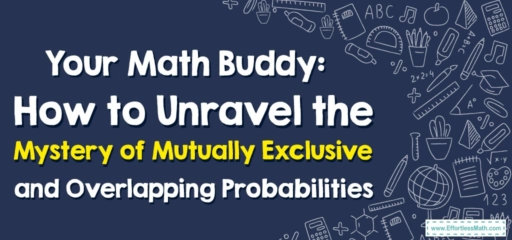# Your Math Buddy: How to Unravel the Mystery of Mutually Exclusive and Overlapping Probabilities

Hey there, math buddies!

Today, we're about to crack open a couple of cool concepts in the world of probability: mutually exclusive and overlapping events. So, pull up a chair, grab your favorite math notebook, and let's dive in!## 1. Decoding Probability Lingo: Mutually Exclusive and Overlapping Events

Here’s the gist:

• Mutually Exclusive Events: These are events that cannot happen at the same time. Like tossing a coin—it can’t land on heads and tails at once, can it?
• Overlapping Events: These are events that can happen at the same time. Like drawing a card from a deck—it can be a heart and a queen, right?

## 2. Cracking the Code: Calculating Probabilities

So how do we find the probabilities for these event types? Let’s break it down:

### Buddy’s Guide: How to Find Probabilities for Mutually Exclusive and Overlapping Events

#### Step 1: Understand Your Event Type

First off, determine whether your events are mutually exclusive or overlapping. Remember, mutually exclusive events can’t occur simultaneously while overlapping events can.

#### Step 2: Calculate Probability for Mutually Exclusive Events

For mutually exclusive events, the probability of either event happening is the sum of their individual probabilities. So, if you’re calculating the probability of drawing a heart OR a queen from a deck of cards, you’d add the individual probabilities together.

#### Step 3: Calculate the Probability of Overlapping Events

For overlapping events, the probability of either event happening is the sum of their individual probabilities, minus the probability of them both happening. If you’re finding the probability of drawing a heart OR a queen from a deck, you’d add the probabilities of drawing a heart and drawing a queen, then subtract the probability of drawing a queen of hearts.

And there you have it, math buddy! We’ve tackled the fascinating concept of mutually exclusive and overlapping events probability together. Remember, no matter how tricky a concept might seem at first, we can always crack it together. Until next time, keep your math spirits high!

### What people say about "Your Math Buddy: How to Unravel the Mystery of Mutually Exclusive and Overlapping Probabilities - Effortless Math: We Help Students Learn to LOVE Mathematics"?

No one replied yet.

X
30% OFF

Limited time only!

Save Over 30%

SAVE $5 It was$16.99 now it is \$11.99